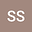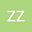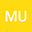A stable time-dependent mesh method to analyze the linear advection-diffusion equation
•••• Zhang Zhengce,
• M. Usman
Xi'an Jiaotong University School of Mathematics and Statistics

Author ProfileZhang Zhengce
Xi'an Jiaotong University School of Mathematics and Statistics
Author ProfileM. Usman
Xi'an Jiaotong University School of Mathematics and Statistics
Author Profile## Abstract

This paper develops the stable adaptive time-dependent mesh scheme for a one-dimensional linear advection-diffusion equation with homogeneous Dirichlet boundary conditions and a sinusoidal initial condition. The aim is to present accurate stable moving nodes finite difference scheme with its stability and convergence. The boundary layer of the flow is exponential therefore difference scheme needs mesh refinement. The moving mesh method analyzes the problem physics and adjusts the mesh according to the problem as it moves nodes in the region of edges. We develop numerical results using four MMPDEs with varying numbers of nodes. A conservative semi-discretization finite difference scheme is used for the spatial derivative and backward Euler difference scheme is employed for the temporal derivative. We have presented five cases in detail to understand the physics of the problem. The proposed moving mesh finite difference method is considerably more efficient than the numerical methods offered in the literature.